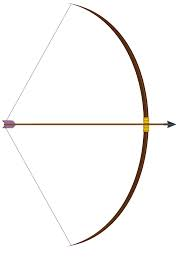# Will Archer A Survive Extended...

Probability Level 5Three archers, A, B, and C, are standing equidistant from each other, forming an equilateral triangle. The chances that A, B, and C successfully hit a target they aim at are $\frac { 1 }{ 3 }$, $\frac { 2 }{ 3 }$, and $\frac { 3 }{ 3 } ,$ respectively.

The three archers will play a survival game. The objective of the game for all players is to kill the other two archers and be the only survivor.

The order of shooting is to be chosen AT RANDOM.

Assuming that all archers will die if he is hit by an arrow aimed at him, and that all archers will make the best possible optimal moves to maximize their chances of winning (surviving), the probability that Archer A wins can be expressed as $\dfrac{a}{b}$ where $a$ and $b$ are co-prime positive integers.

Find the value of $a+b$.

×